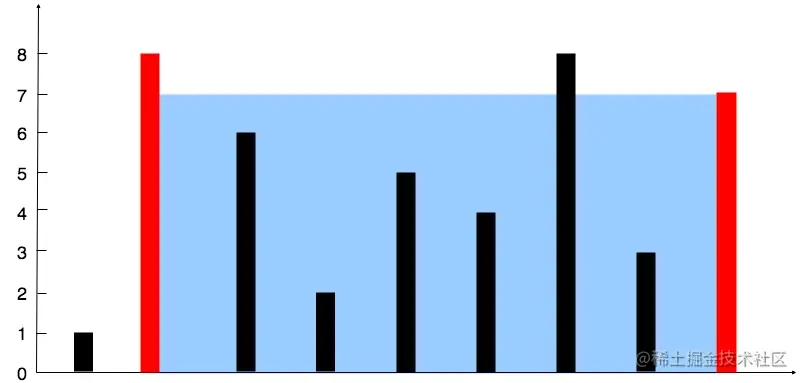# LeetCode 11. 盛最多水的容器｜刷题打卡

295

## 题目描述

Leetcode 链接：11. 盛最多水的容器（medium）``````输入：[1,8,6,2,5,4,8,3,7]

};
``````

• n = height.length
• 2 <= n <= 3 * 104
• 0 <= height[i] <= 3 * 104

## 思路分析

``````var maxArea = function (height) {
let left = 0, right = height.length - 1, maxArea = 0;
while (left < right) {
let currentWidth = right - left,
currentHeight = Math.min(height[left], height[right]);
maxArea = Math.max(maxArea, currentWidth * currentHeight);
if (currentHeight === height[left]) {
left++;
}
else {
right--;
}
}
return maxArea;
};
``````